## The Delta Function Model of a Molecule *

The use of two delta functions allows us to see, to some extent, how atoms bind into molecules. Our potential iswith attractive delta functions at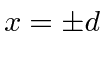. This is a parity symmetric potential, so we can assume that our solutions will be parity eigenstates.

For even parity, our solution in the three regions is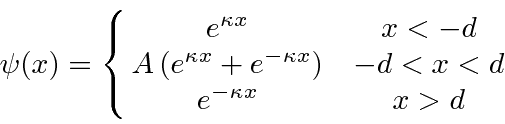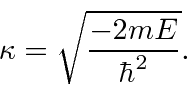Since the solution is designed to be symmetric about, the boundary conditions atare the same as at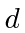. The boundary conditions determine the constant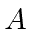and constrain.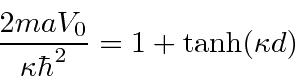This is a transcendental equation, but we can limit the energy.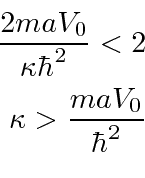Since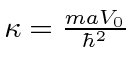for the single delta function, thisis larger than the one for the single delta function. This means thatis more negative and there is more binding energy.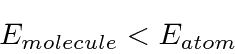Basically, the electron doesn't have to be a localized with two atoms as it does with just one. This allows the kinetic energy to be lower.

The figure below shows the two solutions plotted on the same graph as the potential.Two Hydrogen atoms bind together to form a molecule with a separation of 0.74 Angstroms, just larger than the Bohr radius of 0.53 Angstroms. The binding energy (for the two electrons) is about 4.5 eV. If we approximate the Coulomb potential by with a delta function, settingeV Angstroms, our very naive calculation would give 1.48 eV for one electron, which is at least the right order of magnitude.

The odd parity solution has an energy that satisfies the equation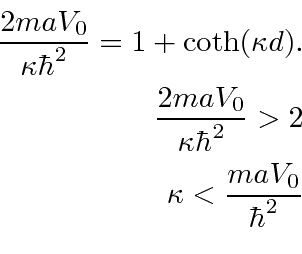This energy is larger than for one delta function. This state would be called anti-bonding.

Jim Branson 2013-04-22